{{announcement.body}}
{{announcement.title}}

# How to Boost Performance With Intel Parallel STL and C++17 Parallel Algorithms

DZone 's Guide to

# How to Boost Performance With Intel Parallel STL and C++17 Parallel Algorithms

### Learn about C++17 parallel algorithms and how to boost performance with this library.

· Performance Zone ·
Free Resource

Comment (0)

Save
{{ articles.views | formatCount}} Views

C++17 brings us parallel algorithms. However, there are not many implementations where you can use the new features. The situation is getting better and better, as we have the MSVC implementation and now Intel’s version will soon be available as the base for `libstdc++` for GCC.

Since the library is important, I’ve decided to see how to use it and what it offers.

## Intro

Until now on my blog, you could read articles where I experimented only with the MSVC implementation of parallel algorithms from C++17.

For example:

However, there’s also another implementation that is worth checking – the one coming with Intel® C++ compiler. I will further refer to it as “Intel’s Parallel STL”.

The library is important as it’s the backbone of future, implementations for GCC and possibly Clang/LLVM.

With Parallel Algorithms added in C++17 you can invoke many algorithms using “execution policies”. So far we have three options:

• `std::execution::seq` - sequential execution, implements `sequenced_policy` type.
• `std::execution::par` - parallel execution, implements `parallel_policy` type.
• `std::execution::par_unseq` - parallel and unsequenced execution, implements `parallel_unsequenced_policy` type.

The vital information is that the execution policies are permissions, not obligations. Each library implementation might choose what can be parallelised and how.

To use parallel algorithms, you need at least forward iterators.

For example:

``````auto myVec = GenerateVector();
std::vector<...> outVec(myVec.size());
std::copy(std::execution::par, myVec.begin(), myVec.end(), outVec.begin());
// ^^^^^^^^^^^^^^^^^^^
// execution policy``````

The above code uses `par` policy. However, implementations might decide not to use parallelism. In copy operations we might be memory bound, so using more threads won’t speed up things.

Developers at Intel built the library to show early support for C++17 Parallel Algorithms. Intel offers many computing and threading solutions, and the library leverages Intel’s famous Threading Building Blocks (TBB).

Here’s the best and concise description of the library:

Parallel STL is an implementation of the C++ standard library algorithms with support for execution policies, as specified in ISO/IEC 14882:2017 standard, commonly called C++17. The implementation also supports the unsequenced execution policy specified in Parallelism TS version 2 and proposed for the next version of the C++ standard in the C++ working group paper P1001R1.

Parallel STL offers efficient support for both parallel and vectorised execution of algorithms for Intel® processors. For sequential execution, it relies on an available implementation of the C++ standard library.

The main repository is located on GitHub. Also, here is the "getting started" document.

The library can work with any C++11 compiler that works with TBB. Also, for vectorization (unsequenced policies) your compiler should support OpenMP 4.0 SIMD constructs.

To use the library you have to include the following headers:

``````#include <pstl/algorithm>  // most of the algorithms
#include <pstl/numeric>    // for reduce, transform_reduce
#include <pstl/execution>  // execution policies
#include <pstl/memory>``````

The library offers four different execution policies:

• `pstl::execution::seq` - sequential execution
• `pstl::execution::unseq` - unsequenced SIMD execution. This is not supported in C++17, but might be available in C++20 (see P0076).
• `pstl::execution::par` - parallel execution
• `pstl::execution::par_unseq` - parallel and SIMD

The use of the library is very similar to the Standard library, instead of `std::execution::par` you just need to use `pstl::execution::par`.

``````auto myVec = GenerateVector();
std::std::sort(pstl::execution::par, myVec.begin(), myVec.end());``````

While C++17 requires Forward Iterators for parallel algorithms, Intel’s library needs stronger condition: Random Access Iterators.

### Backbone Implementation for GCC

The exciting information is that Intel’s implementation serves as a backbone for the upcoming GCC’s (probably GCC 9.0?) version.

Here’s a video from Thomas Rodgers (the developer who brings Intel’s work into GCC):

OpenMP 4.0 is also available under GCC so there’s also a good chance that `unsequenced` policies will also be available in GCC.

Here’s also a thread for LLVM project: [cfe-dev] [RFC] C++17 Parallel STL ∥ a new project.

## How to Install Parallel STL

However the library depends on TBB, so you also have to install in the system.

You can use the following link:

### New Conan Package

Thanks to the support from the Conan Team we added Parallel STL as a Conan package.

To install the library, you can type:

``conan install parallelstl/20181004@conan/stable``

The command will install TBB along with the headers for Parallel STL in your system.

For the test code, apart from Parallel STL I also needed `glm` (OpenGL Math library) and Google Benchmark. Plus of course Visual Studio.

Here's the GitHub repo.

In my previous benchmarks, I’ve used custom code to measure execution. This time I’ve decided to rely on google benchmark - as it offers more options and should give more consistent results.

To manage all dependencies and libraries, I’m using Conan Package Manager.

Conan file - `conanfile.txt`:

``````[requires]
glm/0.9.9.1@g-truc/stable
parallelstl/20181004@conan/stable

[generators]
visual_studio``````

Here’s the command line to install the library (it generates `*.props` file that I can use with my Visual Studio project)

``conan install . -s build_type=Release -if build_release_x64 -s arch=x86_64``

Note about Google benchmark: Currently, the library is not in the Conan center repository, so I’m using a package created by Mateusz Pusz. To use it in your system, you only have to add a remote URL:

``conan remote add conan-mpusz https://api.bintray.com/conan/mpusz/conan-mpusz``

As I know, we should also expect to have google benchmark in the Conan center soon.

I remember that a year or two ago, it was a real pain for me to manage the libraries for my small projects, but with Conan, it’s now straightforward.

## Tests

• Trigonometry - `sqrt(sin*cos)`
• Dot product using large vectors
• Sorting of `vec4`, on the X axis
• Extra example :)

### Trigonometry

As the first example let’s start with something that can be “easily” parallelizable: computing trigonometry functions. The instructions should keep CPU busy, and since there’s no dependency between element computations, then we can see a nice speed increase.

The code:

``````template <typename Policy>
static void BM_Trigonometry(benchmark::State& state, Policy execution_policy)
{
std::vector<double> vec(state.range(0), 0.5);
std::generate(vec.begin(), vec.end(), []() {
return GenRandomFloat(0.0f, 0.5f*glm::pi<float>()); }
);
std::vector out(vec);

for (auto _ : state)
{
std::transform(execution_policy,
vec.begin(), vec.end(),
out.begin(),
[](double v) {
return std::sqrt(std::sin(v)*std::cos(v));
}
);
}
}``````

Benchmark:

``````// MSVC: par and seq only
BENCHMARK_CAPTURE(BM_Trigonometry, std_seq, std::execution::seq);
BENCHMARK_CAPTURE(BM_Trigonometry, std_par, std::execution::par);
BENCHMARK_CAPTURE(BM_Trigonometry, pstl_seq, pstl::execution::seq);
BENCHMARK_CAPTURE(BM_Trigonometry, pstl_par, pstl::execution::par);``````

I’m running all benchmarks with the following params:

``RangeMultiplier(10)->Range(1000, 1000000)->Unit(benchmark::kMicrosecond);``

So that gives us execution for 1000 elements, 10k, 100k and 1000 million elements. The time is shown using microseconds.

Here are the results on the MSVC Compiler, Release x64:Google Benchmark measures the time of execution that happens in the for-loop:

``````for (auto _ : state)
{
// test code...
}``````

It runs our code enough times (number of iterations) to provide stable statistical results. In general the faster the code, the more iteration it needs. That’s why you will see different numbers of iterations near the results.

To compare it easier, here’s a chart with the results for 100k and 1 million elements: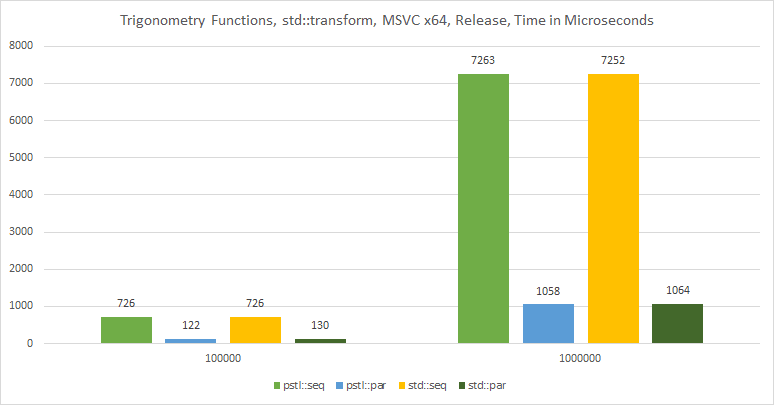On MSVC, the performance is very similar.

My machine has 6 cores, 12 threads. So parallel policies were able to achieve almost 7x speedup.

And also here are the results when compiling under Intel® C++ Compiler 18.0

The code:

``````BENCHMARK_CAPTURE(BM_Trigonometry, pstl_seq, pstl::execution::seq);
BENCHMARK_CAPTURE(BM_Trigonometry, pstl_unseq, pstl::execution::unseq);
BENCHMARK_CAPTURE(BM_Trigonometry, pstl_par, pstl::execution::par);
BENCHMARK_CAPTURE(BM_Trigonometry, pstl_par_unseq, pstl::execution::par_unseq);``````

The results:Intel® C++ Compiler 18.0 doesn’t support Standard Parallel Algorithms, so I only use `pstl::execution` policies. What’s interesting here is that we see a real performance improvement when using unsequenced policies.

For example, for one million elements unsequenced policy is 3x faster than the sequential version. I need to examine the results as they don’t look right. For example, the sequential version is 2x slower than the sequential version on MSVC.

### Dot Product (Using `transform_reduce`)

The previous tests used `transform` that can be easily parallelized. However, how about more complex parallel invocation?

For example, using reduce operation - which is another core component of parallel algorithms.

Reduction needs to work with several steps to compute a single result of the input range of elements. `std::reduce` is a form of `std::accumulate`.

C++ offers a fused version of two algorithms: reduce and transform. That way elements in the container are firstly transformed using some unary operation, and then the results are reduced (“summed”) into a single value.

We can use the pattern to compute the dot product of two large vectors. In the first step - transform - we’ll multiply components from the vector, and then add the results together.

``A dot B = a1*b1 + ... + an*bn;``

C++ code:

``````template <typename Policy>
static void BM_DotProduct(benchmark::State& state, Policy execution_policy)
{
std::vector<double> firstVec(state.range(0));
std::vector<double> secondVec(state.range(0));

//initialize vectors with random numbers
std::generate(pstl::execution::par,
firstVec.begin(), firstVec.end(),
[]() { return GenRandomFloat(-1.0f, 1.0f); });
std::generate(pstl::execution::par,
secondVec.begin(), secondVec.end(),
[]() { return GenRandomFloat(-1.0f, 1.0f); });

for (auto _ : state)
{
double res = std::transform_reduce(execution_policy,
firstVec.cbegin(), firstVec.cend(),
secondVec.cbegin(), 0.0,
std::plus<double>(),
std::multiplies<double>());
benchmark::DoNotOptimize(res);
}
}``````

Results on MSVC:The chart:And here are the results when compiled using Intel® C++ Compiler: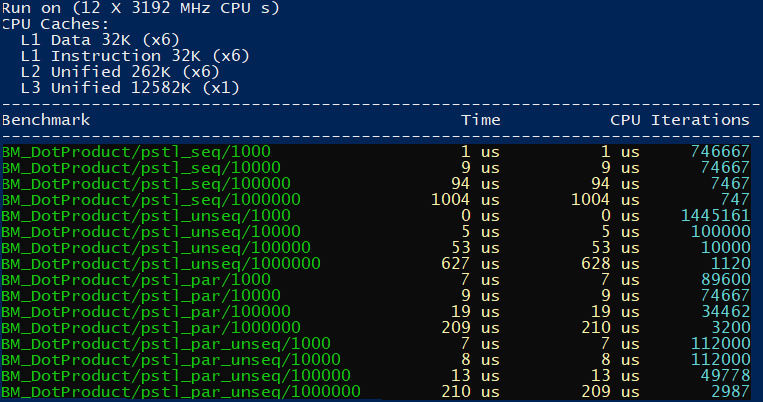### Sorting

Another more complex pattern for parallel execution is sorting.

I’ll be creating `vec4` objects, and I’d like to sort them on the X-axis.

We can use the following code for the benchmark:

``````template <typename Policy>
static void BM_SortPoints(benchmark::State& state, Policy execution_policy)
{
std::vector<glm::vec4> points(state.range(0), { 0.0f, 1.0f, 0.0f, 1.0f });
std::generate(points.begin(), points.end(), []() {
return glm::vec4(GenRandomFloat(-1.0f, 1.0f),
GenRandomFloat(-1.0f, 1.0f),
GenRandomFloat(-1.0f, 1.0f), 1.0f);
}
);

for (auto _ : state)
{
std::sort(execution_policy, points.begin(), points.end(),
[](const glm::vec4& a, const glm::vec4& b) {
return a.x < b.x;
}
);
}
}``````

The results from MSVC:The chart: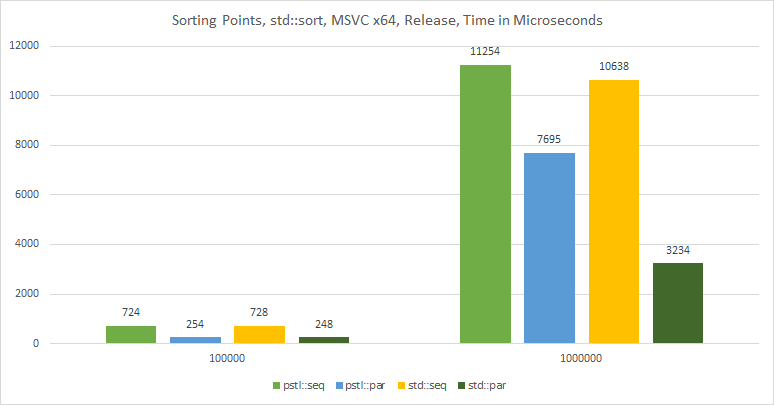And from the Intel® C++ Compiler:The above example shows only `seq` and `par` executions as `unsequenced` policies are not supported in this case.

### Extra — Counting and Zip Iterators

When I dig into the sources of Parallel STL I’ve noticed two potentially useful helpers: zip and counting iterators.

They are defined in `#include <iterators.h>` header file, and only reference to TBB’s iterators:

The iterators are handy when you’d like to access several containers in one parallel algorithm.

Here’s an example where I compute the profit from orders:

``profit = price * (1.0f - discount)*quantity``

And the code:

``````std::vector<double> prices(VecSize);
std::vector<unsigned int> quantities(VecSize);
std::vector<double> discounts(VecSize);

std::for_each(execution_policy,
pstl::counting_iterator<int64_t>(0),
pstl::counting_iterator<int64_t>(VecSize),
[&prices, &quantities, &discounts](int64_t i) {
prices[i] = GenRandomFloat(0.5f, 100.0f);
quantities[i] = GenRandomInt(1, 100);
discounts[i] = GenRandomFloat(0.0f, 0.5f); // max 50%
}
);

// compute profit:
std::vector<double> profit(VecSize);
std::transform(execution_policy,
pstl::counting_iterator<int64_t>(0),
pstl::counting_iterator<int64_t>(VecSize), profit.begin(),
[&prices, &quantities, &discounts](int64_t i) {
return (prices[i] * (1.0f - discounts[i]))*quantities[i];
}
);``````

Currently, the iterators are TBB/Parallel STL specific, so they are not fully conformant with STL (missing default constructor for iterators).

I’ve also created a benchmark for the above example:

``````template <typename Policy>
static void BM_CountingIter(benchmark::State& state, Policy execution_policy)
{
const auto VecSize = state.range(0);
std::vector<float> prices(VecSize);
std::vector<unsigned int> quantities(VecSize);
std::vector<float> discounts(VecSize);
std::vector<float> profit(VecSize);

std::for_each(execution_policy,
pstl::counting_iterator<int64_t>(0),
pstl::counting_iterator<int64_t>(VecSize),
[&prices, &quantities, &discounts](int64_t i) {
prices[i] = GenRandomFloat(0.5f, 100.0f);
quantities[i] = GenRandomInt(1, 100);
discounts[i] = GenRandomFloat(0.0f, 0.5f); // max 50%
}
);

for (auto _ : state)
{
std::transform(execution_policy,
pstl::counting_iterator<int64_t>(0),
pstl::counting_iterator<int64_t>(VecSize), profit.begin(),
[&prices, &quantities, &discounts](int i) {
return (prices[i] * (1.0f - discounts[i]))*quantities[i];
}
);
}
}``````

Here are the results when running on Intel® C++ Compiler: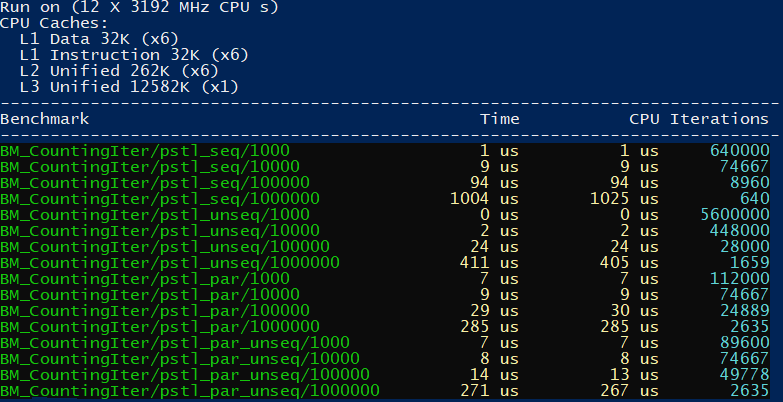In the above test run we can see that `unseq` yields 2.5X speedup over the sequential version. However in `par_unseq` we don’t get much extra speed over the `par` version.

In C++20 we might have a similar counting iterator that will be implemented along with the Ranges.

## Summary

It was an interesting experiment!

In most cases on MSVC TBB implementation and internal Visual Studio’s approach performed similarly on my Windows machine.

Intel offers unsequenced policies so it would be a good option to check Parallel STL on Clang and GCC (as they support TBB and also `SIMD` constructs with OpenMP).

I look forward to getting the standard algorithm support on GCC and Clang.

Below you can see a summary of the differences between Parallel STL and Standard C++.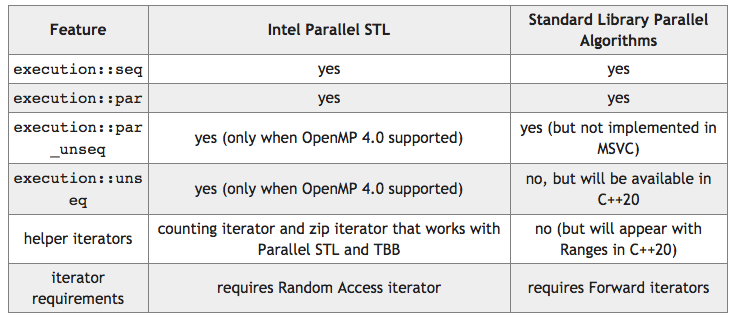Have you played with Intel Parallel STL or TBB?

### Special Thanks to the Conan Team

I’m grateful to the Conan Team for sponsoring the article and also providing the real value - a fresh Intel Parallel STL Conan package. You can easily use it in your projects.

Topics:
parallel algorithms ,c++ 17 ,c++ ,performance ,tutorial ,performance optimization ,gcc

Comment (0)

Save
{{ articles.views | formatCount}} Views

Published at DZone with permission of

Opinions expressed by DZone contributors are their own.